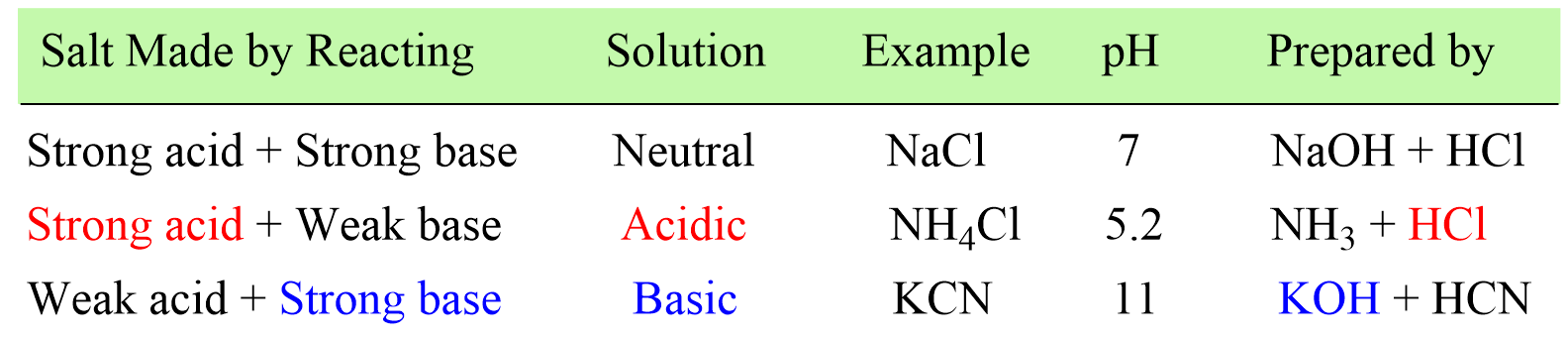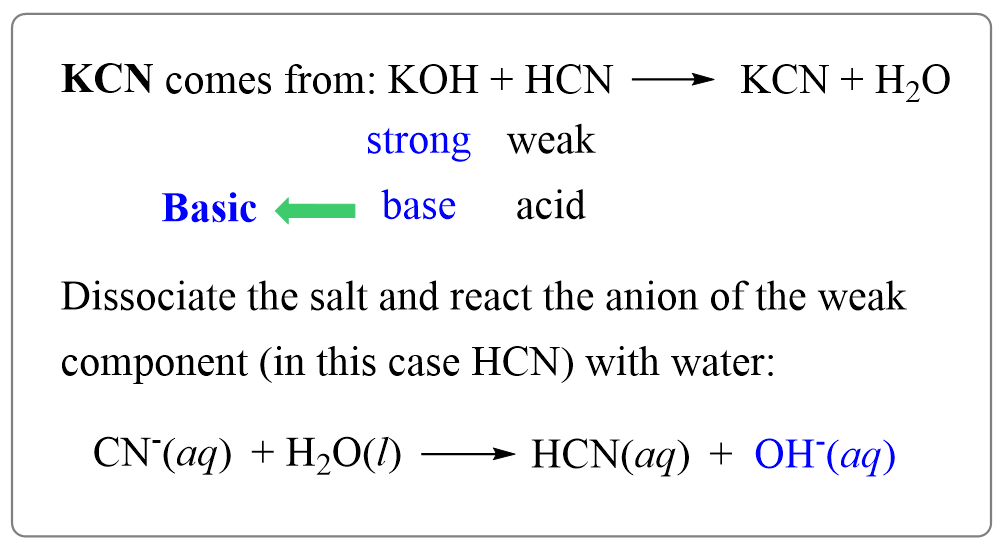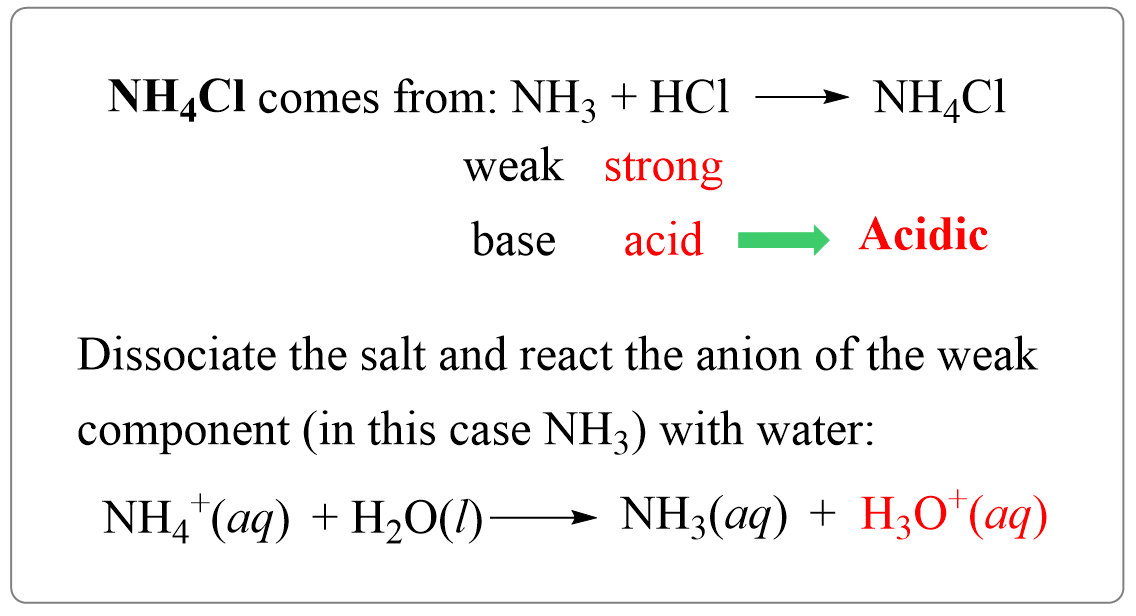## General Chemistry

First, recall that a salt is an ionic compound formed by the reaction between an acid and a base where the acid provides the anion, and the base brings the cation to the salt.

HCl(aq) + NaOH(aq) →  NaCl(aq) + H2O(l)

When the salt is dissolved in water, it dissociates into ions, and depending on which of these ions reacts with water, an acidic or a basic solution is formed. In other words, the pH of the salt solution depends on the type of the acid and the base it is prepared from.

A good shortcut to figuring out if the salt is acidic or basic, is to identify the “strong component” in it.# Salts that Yield Basic Solutions

For example, potassium cyanide (KCN) is prepared from a strong base (KOH) and weak acid (HCN), and therefore, its aqueous solution will be basic.

You can also write the reaction if in doubt:

KOH + HCN → KCN + H2O

So, we have a shortcut to determine if the solution will be basic or acidic, but how does that work?

For this, identify its component ions – K+ and CN:

Now, out of these two, the ion of the weak component (acid or base) that forms the salt is strong enough to react with water. Remember, the stronger the acid, the weaker its conjugate base, and the stronger the base, the weaker its conjugate acid.

You can read more about the acid-base strength and their correlation here.

So, the ion of the weak component (the acid or the base making the salt) reacts with water and as a result of this reaction, either H3O+ or OH ions are formed making the solution acidic or basic respectively.

CNis the anion component (conjugate base) of HCN which is the weaker component of KCN, while K+ is the cation of a strong base KOH. Therefore, we will write the reaction of CN ion with water where it acts as a base removing a proton from water.

CN(aq)  + H2O(l) → HCN(aq)  +  OH(aq) (basic)

So, the reaction of the salt, or more accurately one of the ions of the salts, produces a hydroxide ion which makes the solution basic.

In general, remember that bases are negatively charged or at least have a lone pair of electrons that abstracts a proton from the acid, which in this case is the water:

Writing the reaction of the weak component ions is important in calculating the pH of the salt solution. However, if you only need to tell if the solution will be acidic or basic, identify the strong component making up the salt.# Salts that Yield Acidic Solutions

Ammonium chloride (NH4Cl) is the salt of ammonia (NH3) and hydrochloric acid (HCl) and it yields an acidic solution. So, let’s follow what we have discussed earlier and see why it produces an acidic solution.

Step 1. Determine the acid and base that the salt is prepared from:

NH3 + HCl → NH4Cl

It is made of a weak base ammonia and a strong base HCl, and therefore, the solution is going to be acidic. And that’s it – we determined if the solution is basic or acidic. Let’s add step two which is going to be very important for calculating the pH of a salt solution.

Step 2. Dissociate the salt to identify the ion of the weaker compoenent.

NH4Cl → NH4+ + Cl

NH4+ is the ion with a weaker component (it is the conjugate acid of a weak base ammonia NH3), so it is going to react with water producing hydronium ion which makes the solution acidic:

NH4+(aq)  + H2O(l) → NH3(aq)  +  H3O+(aq)  (acidic)

In summary, this is what we did:# Salts that Yield Neutral Solutions

The table salt NaCl found also in physiological solutions has a negligible effect on the pH of the solution.

Take a few minutes and see if you can figure out why it does not alter the pH.

Like we did before, determine the acid and the base that make the salt. In this case, it is HCl and NaOH,  which make a strong acid a strong base:

NaCl+(aq) → Na+(aq) + Cl(aq)

The salts of strong acids and bases produce neutral solutions.

The reason for this is that the cation and anion of a salt derived from a strong acid and a strong base, do not react with water and thus, no H3O+ or OH is generated.In the next post, we will use this strategy to calculate the pH of salt solutions.

Check Also

1685282694